# Quick Math Solver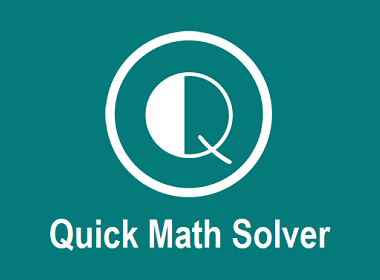10 Math Problems officially announces the release of Quick Math Solver, an android app on the Google Play Store for students around the world.

What is a Quick Math Solver?

Quick Math Solver is a mobile web application for school-level mathematics. It is especially helpful for the students of grades V to X. It gives step-by-step solutions to the mathematical problems currently from the chapters ARITHMETIC, ALGEBRA, MENSURATION, GEOMETRY, STATISTICS, and MATRICES. And, will be added more in upcoming days.

Currently, you can solve the following math problems by using this application.

FROM ARITHMETIC (NUMBERS):

ü Simplify by using the BODMAS rule

ü Check PRIME or COMPOSITE number

ü List the FACTORS of a number

ü Find the PRIME FACTORS by DIVISION method

ü Find PRIME FACTORS by FACTOR TREE method

ü Find HCF by definition method

ü Find HCF by prime factor method

ü Find HCF by division method

ü Find LCM by definition method

ü Find LCM by prime factor method

ü Find LCM by division method

FROM ALGEBRA:

ü FACTORIZE the algebraic expression

ü SIMPLIFY the algebraic expression

ü Find the HCF/LCM of given algebraic expressions

ü SOLVE the algebraic equations

ü SOLVE one linear equation in one variable

ü SOLVE simultaneous linear equations by elimination method

ü SOLVE quadratic equation by factorization method

ü SOLVE quadratic equation by using formula

ü SOLVE rational algebraic equation

FROM MENSURATION

ü PLANE FIGURE (2 Dimensional): Find the AREA, PERIMETER, etc. of Triangle, Right Angled Triangle, Quadrilateral, Square, Rectangle, Parallelogram, Rhombus, Trapezium, Circle, etc.

ü SOLID FIGURE (3 Dimensional): Find the LATERAL SURFACE AREA, CURVED SURFACE AREA, TOTAL SURFACE AREA, VOLUME, etc. of Cube, Cuboid, Sphere, Cylinder, Cone, Prism, Pyramid, etc.

FROM GEOMETRY

ü Find unknown angles from ANGLE AND PARALLEL LINES

ü Find unknown angles from TRIANGLES

ü Find unknown angles from Circles

FROM STATISTICS:

ü FIND MODE

ü FIND RANGE

ü FIND MEAN

ü FIND MEDIAN

ü FIND QUARTILES

ü FIND MEAN DEVIATION FROM MEAN

ü FIND MEAN DEVIATION FROM MEDIAN

ü FIND QUARTILE DEVIATION

ü FIND STANDARD DEVIATION BY DIRECT METHOD

FROM MATRICES:

ü FIND TRANSPOSE

ü FIND DETERMINANT

ü FIND INVERSE

Here are some of the SCREENSHOTS of the App: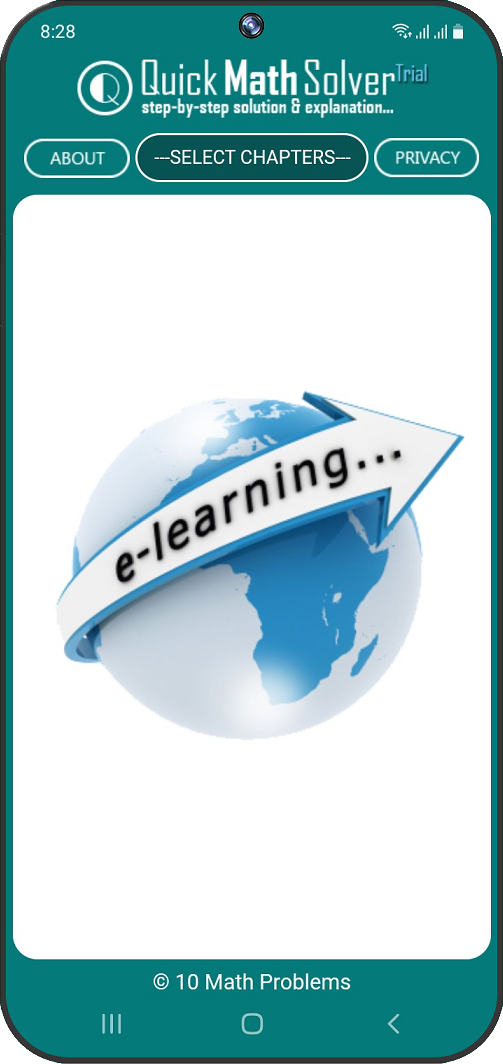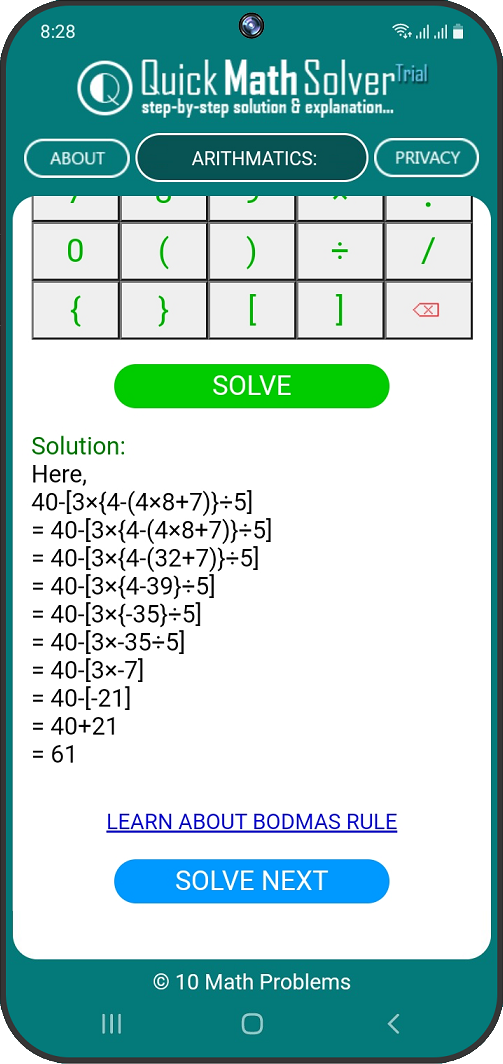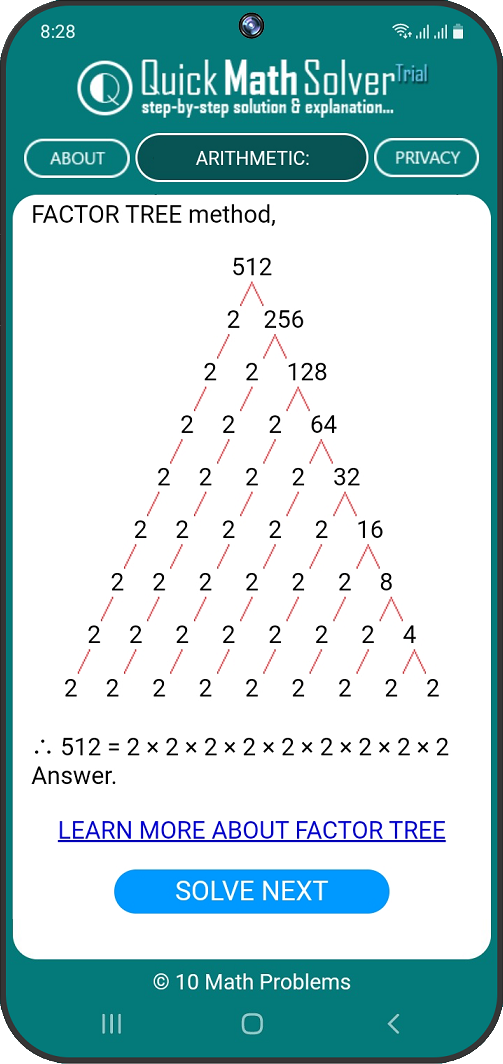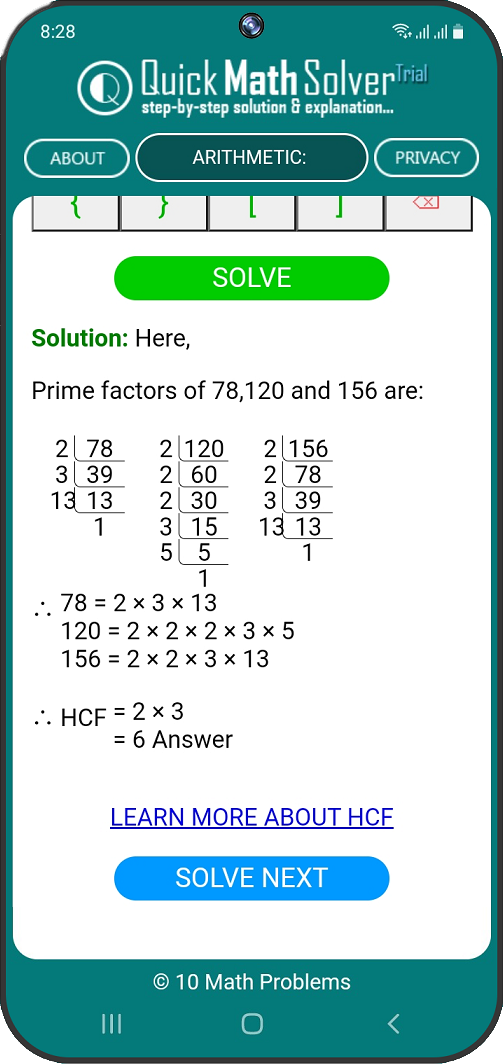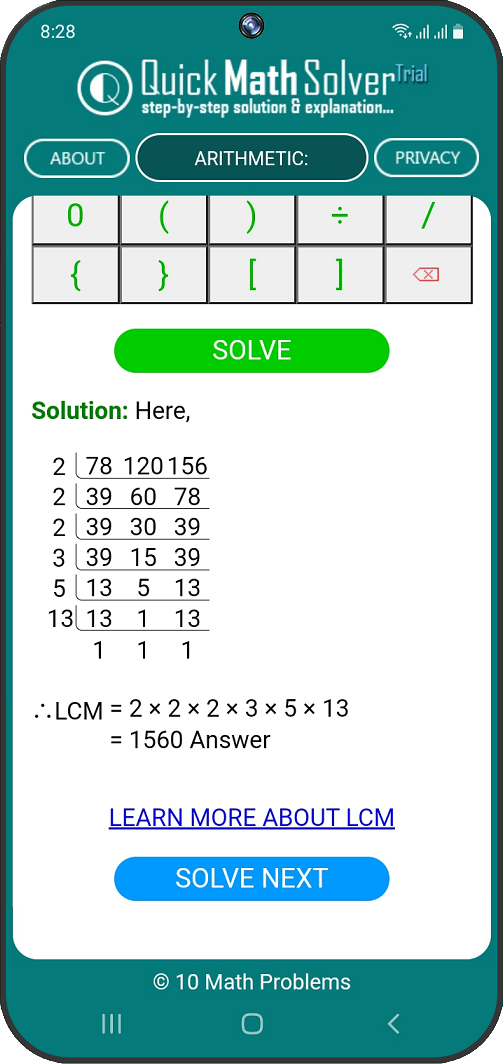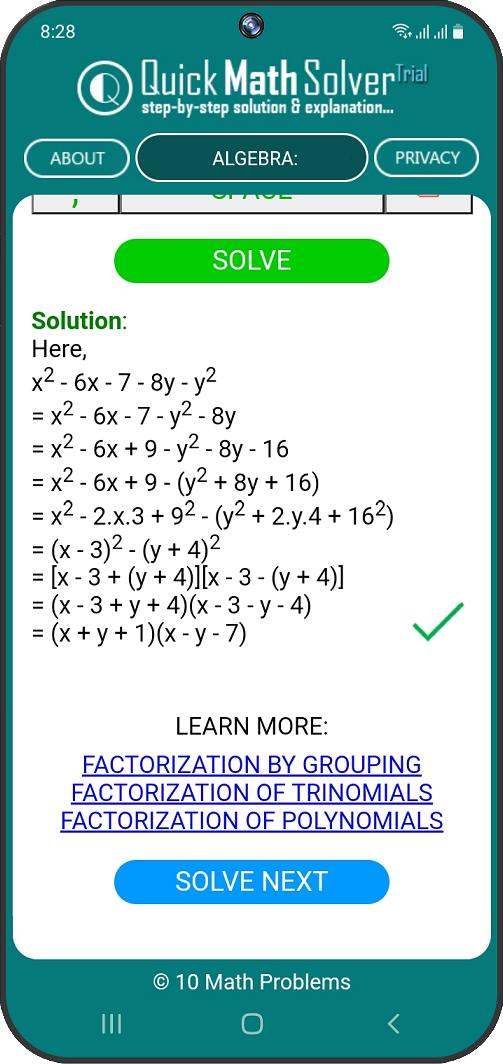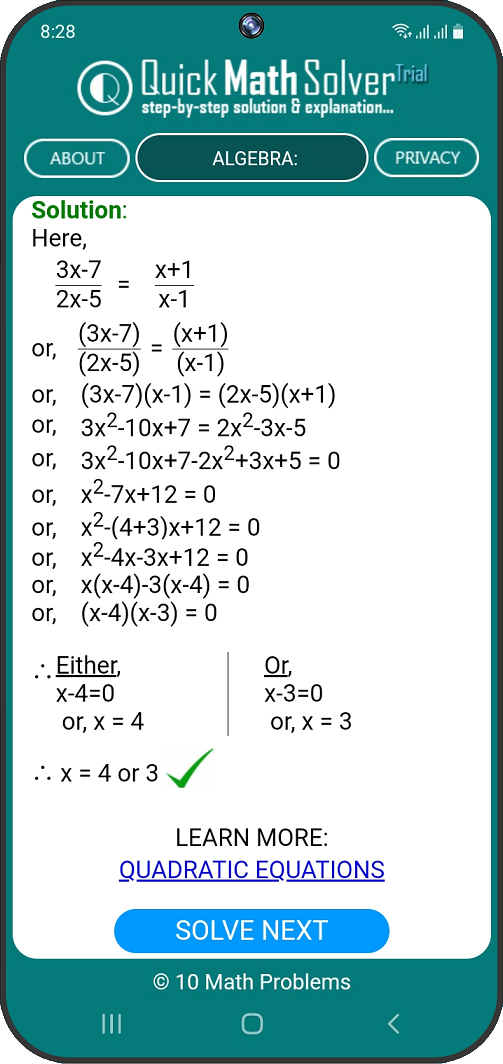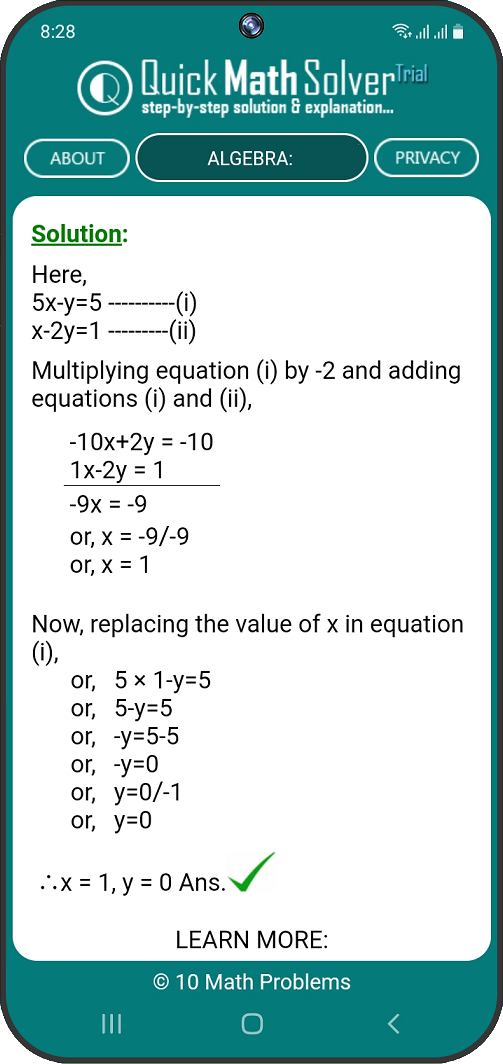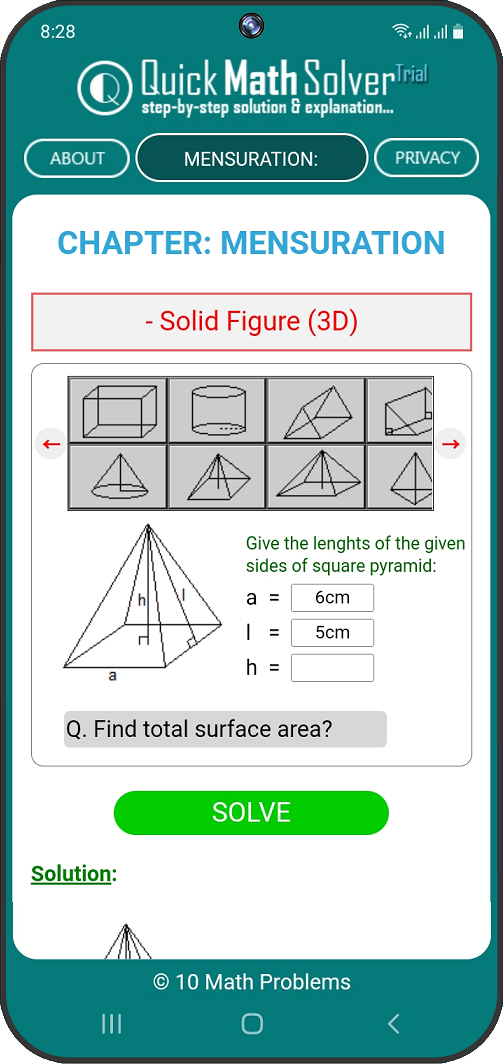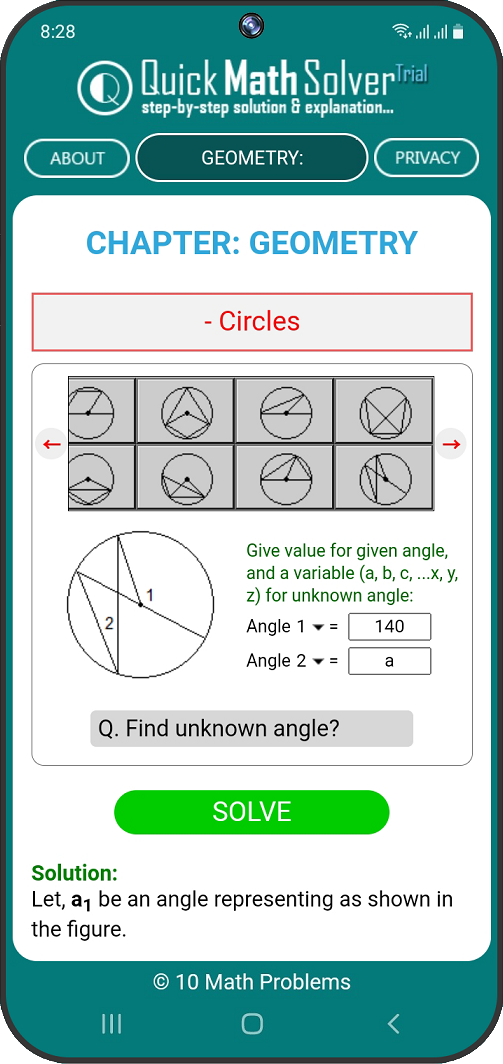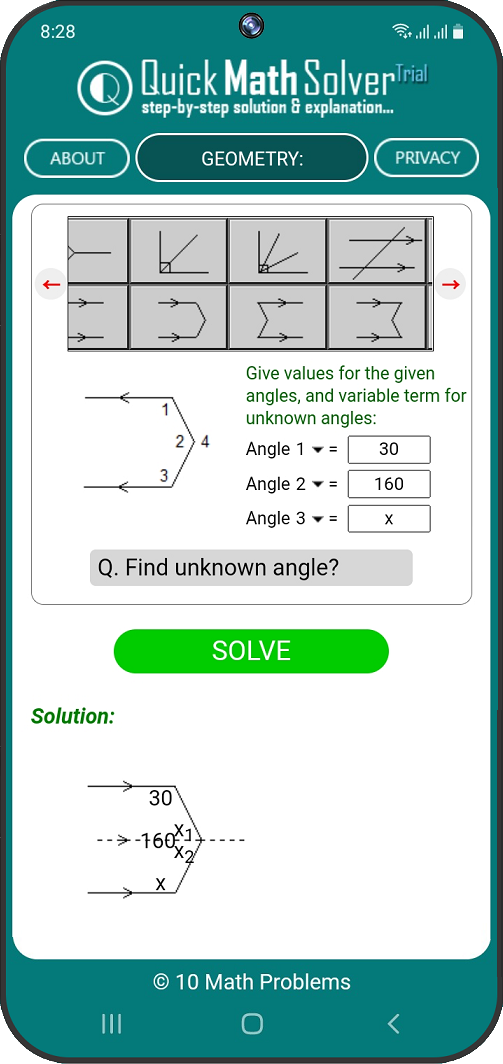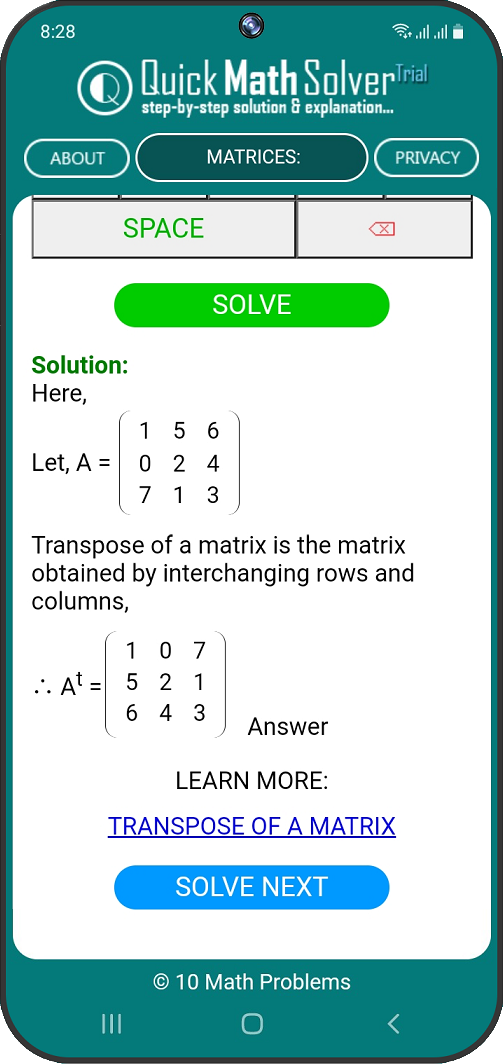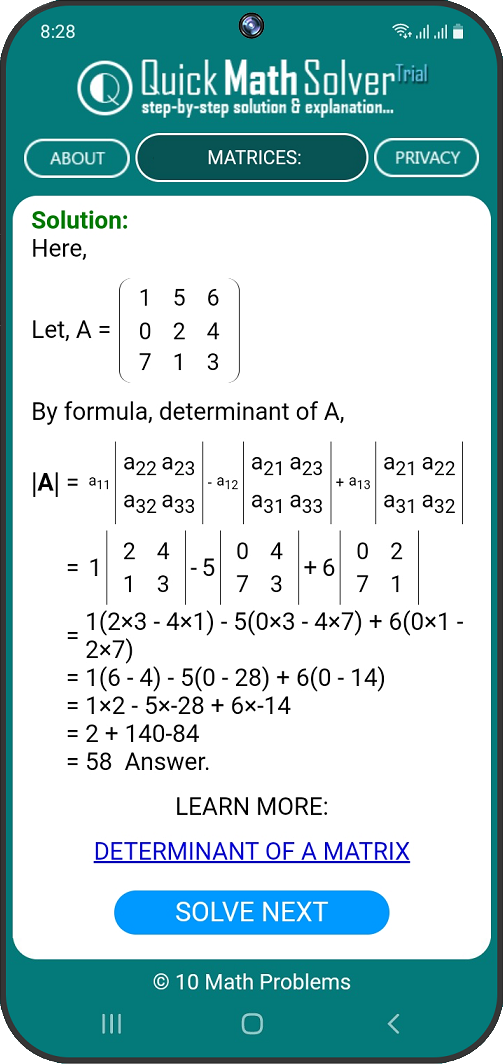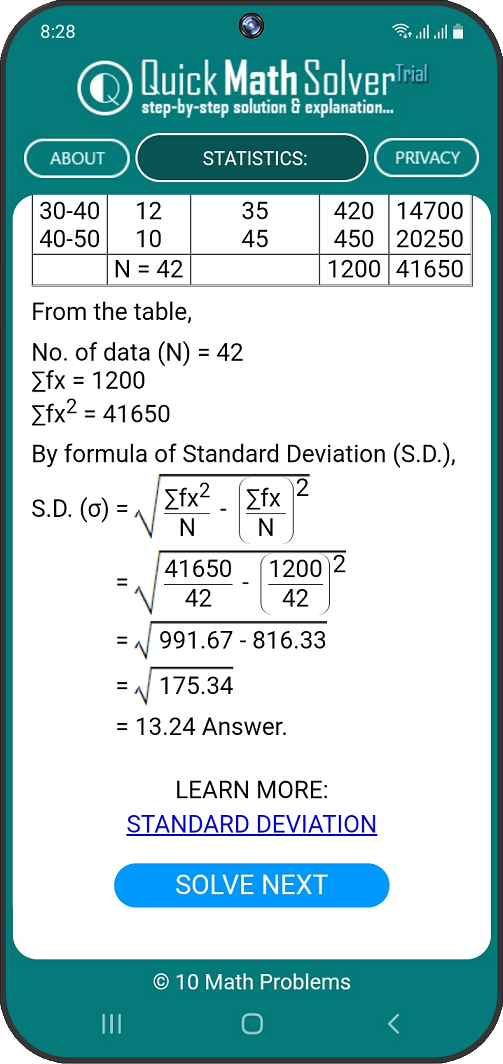You can Install the Quick Math Solver App on your android phone from the Google Play Store.

If you like the APP, please add a REVIEW COMMENT and give a STAR RATING in the Google Play Store.